# Hidden Markov Models: The Backwards Algorithm

I just finished working on LEARNINGlover.com: Hidden Marokv Models: The Backwards Algorithm. Here is an introduction to the script.

Suppose you are at a table at a casino and notice that things don’t look quite right. Either the casino is extremely lucky, or things should have averaged out more than they have. You view this as a pattern recognition problem and would like to understand the number of ‘loaded’ dice that the casino is using and how these dice are loaded. To accomplish this you set up a number of Hidden Markov Models, where the number of loaded die are the latent variables, and would like to determine which of these, if any is more likely to be using.

First lets go over a few things.

We will call each roll of the dice an observation. The observations will be stored in variables o1, o2, …, oT, where T is the number of total observations.

To generate a hidden Markov Model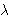(HMM) we need to determine 5 parameters:

• The N states of the model, defined by S = {S1, …, SN}
• The M possible output symbols, defined by= {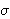1,2, …,M}
• The State transition probability distribution A = {aij}, where aij is the probability that the state at time t+1 is Sj, given that the state at time t is Si.
• The Observation symbol probability distribution B = {bj(k)} where bj(k) is the probability that the symbolk is emitted in state Sj.
• The initial state distribution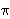= {i}, wherei is the probability that the model is in state Si at time t = 0.

The HMMs we’ve generated are based on two questions. For each question, you have provided 3 different answers which leads to 9 possible HMMs. Each of these models has its corresponding state transition and emission distributions.

• How often does the casino change dice?
• 0) Dealer Repeatedly Uses Same Dice
• 1) Dealer Uniformly Changes Die
• 2) Dealer Rarely Uses Same Dice
• Which sides on the loaded dice are more likely?
• 0) Larger Numbers Are More Likely
• 1) All Numbers Are Randomly Likely
• 2) Smaller Numbers Are More Likely
How often does the casino change dice?
Which sides on
are more likely?
 (0, 0) (0, 1) (0, 2) (1, 0) (1, 1) (1, 2) (2, 0) (2, 1) (2, 2)

One of the interesting problems associated with Hidden Markov Models is called the Evaluation Problem, which asks the question “What is the probability that the given sequence of observations O = o1, o2, …, oT are generated by the HMM. In general, this calculation, p{O |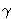}, can be calculated by simple probability. However because of the complexity of that calculation, there are more efficient methods.

The backwards algorithm is one such method (as is the forward algorithm). It creates an auxiliary variablet(i) which is the probability that the model has generated the partially observed sequence ot+1, …, oT, where 1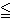tT. This variable can be calculated by the following formula:t(i) =j = 1 to N(t+1(j) * aij * bj(ot+1))

We also need thatT(i) = 1, for 1iN.

Once we have calculated thet(j) variables, we can solve the evaluation problem by p{O |}i = 1 to N1(i)

There is more on this example at LEARNINGlover.com: Hidden Marokv Models: The Backwards Algorithm.

Some further reading on Hidden Markov Models:

# Understanding Bayes’ Theorem

I’ve finished a script that helps understand Bayes’ Theorem.

If we have a set of mutually exclusive (aka non-overlapping) sets Bi for i{0, 1, 2, …, n} for some integer n, then the union of these sets forms a sample space. Lets call the sample space S. Suppose that we also have some set (also known as an event) A which is also a subset of S. Bayes’ Theorem considers the probability that one of these mutually exclusive events (one of the Bi‘s) caused the observed event (A).

This probability can be calculated by the formula

 Pr(Bj | A) = Pr(Bj) Pr(A | Bj)Pr(Bi) Pr(A | Bi)

The theorem helps us determine the the probability of the event Bj given A, or in more plain English, the probability that the event Bj is the cause that gives rise to the observed event A. The numerator is given by the product of of the probability of the causal event (Pr(Bj) times the conditional probability of the observed event given the causal event (Pr(A | Bj)). This numerator could be replaced by its equivalent statement of the set A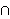Bj. Likewise, the denominator the sum (over all the causal events) of the probaility of each causal event times the conditional probability of the observed event given that particular causal event. Each term in this denominator could be replaced b its equivalent staetment ABi, which when summed give the total probability of A because each pair of the Bj‘s is mutually exclusive. So we are able to replace the probability of A withPr(Bi) Pr(A | Bi) because of the fundamental law of probability.

An example that would use Bayes’ Theorem is analyzing the results of an election. The set of mutually exclusive events could be membership in a political party (Democrat, Republican, or Independent). The observed event could be the election of an individual. And the conditional distributions could be the percentage of each party that voted for this individual. If we want to calculate how significant each party was to the individual’s election, we’d use Bayes’ Theorem.

The script I’ve written to help understand Bayes’ Theorem works as follows:
– A set of mutually exclusive sets is randomly generated (the number of sets also varies). These sets are called Bi for i(0, …, n}.
– A set A is randomly generated from the union of the Bi‘s.
– A table is displayed showing:
Pr(Bi) for each i on line 1.
Pr(A | Bi) for each i on line 2.

– The user is given the option to select which of the mutually exclusive sets they would like to use to calculate the probability that this set caused the event A.
– Once a set is chosen, the user clicks the “Calculate Conditional” button and Bayes’ Theorem gives the result.
– If the “show work” checkbox was checked, then the steps used in this calculation are also shown.
– All work is done using fractions to give an idea of where the numbers come from.

Other Blogs that have covered this topic:
Better Explained
Bayes’ Theorem-qed

# K-Means ClusteringI have now uploaded my K-Means Clustering Script. The script generates a set of random numbers (as ordered pairs) and asks the user how many clusters we should divide the numbers into and a maximum number of iterations to go through before we stop.

One of the largest problems that we face today is understanding data. Before we even get to the point of trying to interpret what the data means and making decisions based on that data there is often a problem with the general amount of data. Clustering algorithms seek to solve this problem by defining some notion of similarity and using that notion to group the data into sets or ‘clusters’, where two elements belong to the same cluster if they are considered similar. Once elements are placed into clusters, we can analyze this (generally smaller) set of clusters instead of the entire data set, which should help in understanding the data.

Finding an exact solution to this problem is computationally difficult. Instead, we can approximate a solution rather quickly using the k-means clustering algorithm. This algorithm attempts to separate a given data set into a user specified (k) number of groups. The k signifies the number of clusters that we will generate. The algorithm works by initially selecting k elements of the data to serve as the “center” of each cluster. Every element of the data is then compared to each cluster center and assigned to the cluster with the closest cluster center. Once every element in the data is assigned to a cluster, the cluster centers may have changed. So the next step is to measure the elements inside each cluster and determine the new cluster center. The process of assigning elements to (new) clusters and determining (new) cluster centers is repeated until either no element changes cluster or we have reached some maximum number of iteration that the user specifies that they do not want to exceed.

K-Means Clustering can be thought of as an algorithm in the area of unsupervised machine learning. Machine learning is a field of artificial intelligence that focuses on computer programs that have the ability to learn without being explicitly programmed. Unsupervised machine learning seeks to make interpret data without any knowledge of what a “correct” interpretation is. In comparison, supervised machine learning algorithms are useful for data that has been separated into categories. These algorithms generally divide the data into a training set and a test set and seek to produce a function that agrees with the results on the training set.

# New Years is a LEARNINGlover Thing!

Starting this web site has served as the perfect opportunity to unwind. In particular, two blogs I wrote recently have served different purposes. The first was “The Degrees of Consciousness of a Black Nerd“, where I spoke about many of the things I think about being who I am and relating the the (somewhat unique) set of people that I communicate with on a daily basis. The other was what I’ve been working on since mid December. Its the blog entry I wrote on Sudoku and the Sudoku program that I wrote last month using the Dancing Links Algorithm. Since originally writing that, I’ve updated the program with a lot of Sudoku problems, as well as two types of “hints”. One generates the “possibilities matrix” which basically just shows what is possible for each cell. The other scans the possibilities matrix and searches for isolated cells (cells where some number can only go in one row/column/subgrid). Both those additions were extremely fun and provided a nice opportunity to program in my spare time.

So I’ve been thinking about these two things and how much I enjoyed the two, but for different reasons. The Degrees of Consciousness of a Black Nerd brought much attention on facebook where the idea was both accepted and rejected and I was able to explain the ideas further and hear similar stories from others who had similar experiences. Sudoku, on the other hand hasn’t generated as much conversation. A few friends have told me that they liked the program, but I don’t know of too many people outside of myself using it. That’s OK. I didn’t really create the program for publication, moreso because I enjoy Sudoku and took it as a challenge to write a program to solve a puzzle.

That being said, I’ve been thinking about some other things that I would like to do – hopefully they’ll be more than just my opinion, but have some programming, operations research, or mathematical context to them as well. But here’s a list of things that I want to write about in the near future.

• Connections between math and football (or sports in general). Anybody who knows me knows that I’m a huge sports fan. At other sites, I’ve posted stuff on QB rating systems and the flaws/inaccuracies in them. Part of me would like to look further into this stuff and either do a comparison, create a new rating system, or just try to understand (explain) different scouting metrics, particularly for QBs.
• I wrote the Sudoku program, but that’s a well studied problem so it was easy to find research that helped me to understand other stuff. I also studied some problems in Ramsey Theory that can be represented by exact covering algorithms and it would be interesting to try to represent these as exact cover problems and to try to use the Dancing Links algorithm to solve these problems as well, maybe to find a comparative analysis to benchmarks.
• One of the things I’ve picked up on lately is machine learning. While I’ve added flash cards on Bayesian Networks, I would like to add some programs on things like k-means clustering.
• I added my sorting algorithms a few weeks ago, but would like to also add something on data structures (arrays, linked lists, trees, heaps, hash tables, etc). In undergrad this was called the class that weeded people out of computer science majors, so doing something on this type of stuff I think could be helpful to those who want to understand it better.
• I have been in the process of writing a linear programming (Simplex) implementation for a while. I would like to get back to that to allow for a solver that can do at least simple algorithms.
• I’ve written a few drafts that connect math to different areas that I’m interested in (music, sports, philosophy, religion, etc), but I need to find a better way to present the stuff because as they’re currently stated, this stuff can easily be misinterpreted.

The beautiful thing about this site is that I’m not constrained by any advisor or boss or deadlines. Its more of a how am I feeling right now kind of a thing and these are the things I’m feeling right now. So this list is kind of my “New Years Resolutions” for LEARNINGlover.com, and while I reserve the right to change my priorities any time I feel that something else deserves my attention more, these are the things I’m planning to spend time on in the next few weeks.